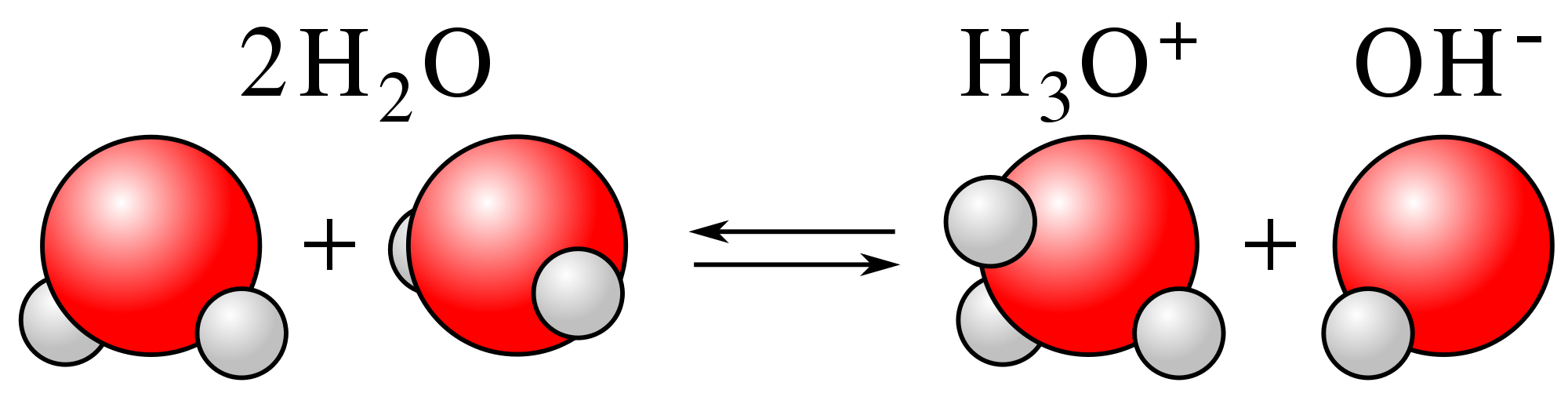Ravela Da Cruz

HS-PS1-2

# Water Ionization Constant Study Guide

Kw in chemistry represents the equilibrium constant when the water goes through the self-ionization process.

## INTRODUCTION

Pure water or distilled water is amphoteric in nature. This means that in its pure state, water goes through the self-ionization process. Self-ionization in this case, means that water molecules donate and accept protons to form H3O+ and OH- ions. The former hydronium ion acts as a strong acid, the latter hydroxide ion is a strong base.

When they come together, they form a water molecule ion. Water maintains a state of equilibrium where both hydronium and hydroxide ions are present, but the state of equilibrium leans to the left side of the water molecule equation. Hence, the concentration of these ions is comparatively less in the water.

### HOW TO CALCULATE Kw IN CHEMISTRY?Source

Kw in chemistry is the equilibrium constant when water goes through self-ionization. During the ionization process, Kw can be represented as:

Kw = [H3O+][OH-] or Kw = [H+][OH-]

As discussed earlier, the state of equilibrium for water lies to the left side of the equation, so there is not much change in the concentration of water molecules. For this reason, we do not consider the concentration of water molecules in the equilibrium equation.

At room temperature, which is 25°C, the value of the water ionization constant comes out to be 1.00 X 10-14 mol² dm⁻⁶.

### HOW TO FIND pH FROM Kw?

Now that we have calculated the value of the water ionization constant, let's see if we can derive pH from Kw. Remember that we are calculating the pH of pure water. It is pertinent to note here that in pure water, the concentration of hydrogen ions and hydroxide ions are the same. Now, going back to the equilibrium equation.

Kw = [H+][OH-]

We can rewrite this equation as:

Kw = [H+]²

Now, we have already derived the value of the ionization constant above so that this equation can be rewritten as:

[H]2 = 1.00 X 10⁻¹⁴ mol² dm⁻⁶

Thus, the concentration of hydrogen ions in pure water would be the square root of the Kw constant.

[H+] = 1 X 10⁻⁷ mol² dm⁻⁶.

Now, the pH of water is calculated by the formula:

pH = - log10 [H+]

By substituting the value of [H+] ions in pure water, we can calculate the pH. Therefore,

pH = - log10 [1 X 10⁻⁷]

Hence, the pH of water comes out to be 7.

## CONCLUSION:

• Pure water is amphoteric in nature and goes through a self-ionization process.
• Kw is the equilibrium constant in the self-ionization process.
• To calculate the Kw, we determine the concentration of hydrogen and hydroxide ions in pure water at room temperature.
• The value of Kw at 25°C is 1.00 X 10-14 mol2dm-6.
• We can also calculate ph from Kw.
• Since the concentration of H+ ions and OH- ions in pure water is the same, the concentration of each of these ions is the square root value of Kw.
• By substituting the value of H+ ion concentration, we can derive the value of water's pH, which comes out to be 7 at room temperature.

### FAQs:

1. Why does Kw not include water?

The Kw equation does not include water because the equilibrium lies to the left during self-ionization, so the concentration of water molecules remains unchanged.

2. What does Kw tell you in chemistry?

Kw in Chemistry represents the equilibrium constant when pure water goes through a self-ionization process.

We hope you enjoyed studying this lesson and learned something cool about Water Ionization Constant! Join our Discord community to get any questions you may have answered and to engage with other students just like you! Don't forget to download our app to experience our fun VR classrooms - we promise it makes studying much more fun! 😎

## SOURCES:

1. Kw Chemistry Definition: https://chemdictionary.org/kw-Chemistry/. Accessed 28 Feb 2022.
2. Self-Ionization of Water: https://www.ck12.org/c/chemistry/self-ionization-of-water-kw/lesson/Ion-Product-of-Water-CHEM/.Accessed 28 Feb 2022.